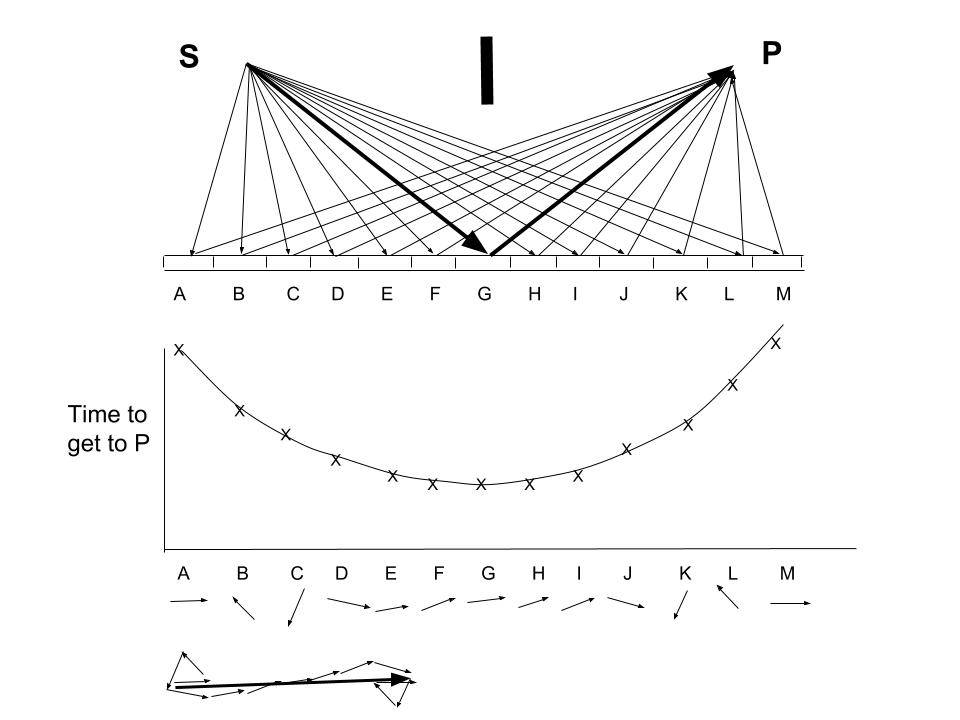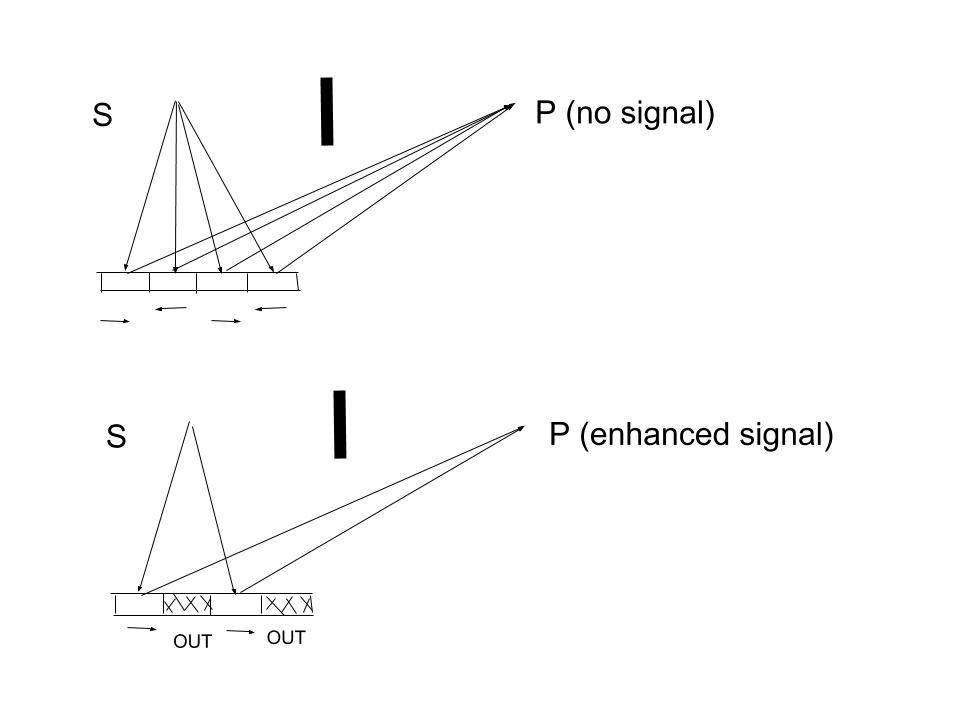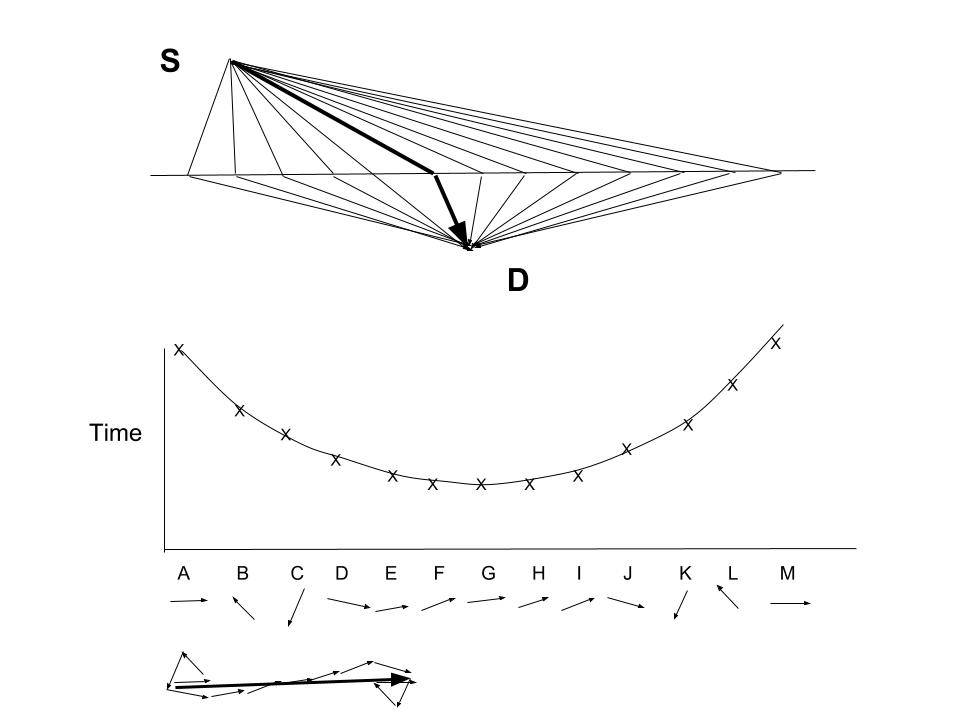# Feynman’s Method of “A Particle Exploring All Possible Paths”

March 29, 2018

1. In the previous post we discussed Professor Feynman’s illustration of how two wave functions (not waves) corresponding to two possible paths for a photon to interfere (constructively and destructively) to produce an oscillating signal; see, “Feynman’s Glass Plate Experiment“.

• It was pointed out that this experimental result is not explainable if the light is treated as an electromagnetic wave.
• More importantly, it showed that a path for a photon is mapped out instantaneously by Nature, as soon as the photon takes off.
• In this post, we will discuss his argument that Nature actually takes into account ALL possible paths, an infinite number of them! This is our key idea behind “nonlocality”, so we will proceed step-by-step to make our case crystal clear.

2.  This discussion is also based on the following figure from Feynman’s book (p 43); see the reference below. Light received at point P due to source S is considered; the direct path from S to P is blocked by a screen placed in between them.

• Everyone is familiar with the “law of light reflection” where the light from A goes to a Point B in a path that is defined by the angle incident being equal to the angle of reflection.• Of course, the time for a photon to get from S to D is a minimum close to the center of the mirror.
• However, Feynman showed that a better picture with more explanatory power is available with the concept of “a photon exploring all paths”. He showed that most possible paths are canceled out and only those paths that lie close to the expected path stated by the Law of Reflection contribute to the final detection probability.

3. In order to illustrate the concept of a photon “exploring all possible paths”, the mirror is divided into sections A through M, and reflection from each section of the mirror is indicated (see the above figure).

• Due to different distances of travel, the time taken for each path varies as shown in the middle figure, and correspondingly the phase varies as shown below that. The final amplitude is given by adding those arrows and is indicated at the bottom of the figure.

4. Just like in adding the contributions from two “waves”, adding the contributions from “wave functions” requires one to take into account the difference in phase angle. Feynman has described this in simple terms, how to add contributions due to many wave functions using vector addition (see pp. 24-35).

• It is evident that the major contribution to the final arrow’s length is made by arrows E through I (from the central part of the mirror), whose directions are nearly the same because the timing of their paths is nearly the same. This also happens to be where the total time is the least indicated by the heavy arrow, which is the expected path from the law of reflection.
• The law of reflection, that we learn at high school, is a simple rule that works. But actually, reflections from each point in the mirror contribute to the signal at P. It is just that most of those contributions cancel out (as shown by the bottom part of the above figure).

5. To prove that even the edge of the mirror does contribute to the signal at P, we chop off most of the mirror, leaving only sections A, B, C on the left. From the above figure, if we add the three arrows due to those three sections, they nearly cancel out. This is why we do not see significant contributions from parts away from the center.

• If we now divide that section (of A, B, C in the above figure) into four equal sections, they of course again cancel out as shown in the top section of the figure below.• But if we now carefully scrape two alternating sections of those four sections (as shown in the bottom figure), then the signals due to the two reflecting sections add up to give an intense signal; see the bottom part of the above figure.

6. This conclusively proves that during normal reflection, parts of the mirror away from the center also contribute to the signal. It is just that most of that signal is canceled out. Thus, for all practical purposes, it is sufficient to just take the reflection from the center part of the mirror (i.e., to use the law of reflection in geometrical optics).

• However, If only the arrows in a particular direction are kept, while the others in opposite direction are removed (by etching the mirror in those places), then a substantial amount of light reflects from a piece of mirror located away from the center, as shown in #5 above.
• That modified section of the mirror is of course now a diffraction grating.

7. Feynman discusses several examples in his book, but let us discuss just one more example to illustrate the point that this method is consistent with the Principle of Causation.

• Here we consider the case of refraction, which had led to causal issues with the “photon as a particle” idea of Newton and Fermat.
• Those who are really interested can read in detail the historical evolution of ideas from Newton through Fermat to Feynman, in the book by Ivar Ekeland (see References below).

8. Figure below shows the refraction of light from a source (S) in the air to a detector (D) placed in water. As in the case of the mirror, we consider all possible paths from S to D and map out the time taken for a photon to reach point D via “different sections” of the water surface.

• The observation of light taking the “time of least time” to reach a detector in the water by changing its path (called “refraction”) was explained by Fermat back in 1657, by taking into account that light travels slower in water than in air; we will discuss this in the next post, “Exploring All Possible Paths” Leads to Fermat’s Principle of Least Time”.
• However, until Feynman came up with his method of “a particle exploring all possible paths”, this phenomenon could not be explained within the “particle picture”.• Once again, most paths away from the optimum path are CANCELLED OUT. The major contributions come from those paths close to the expected arrow indicated by the heavy arrow, and Fermat’s Principle of Least Time is recovered with this “particle representation”.

9. What bothered everyone (including Feynman) about Fermat’s idea is that it seemed to require agency. How could light choose a path? How could it possibly know which path was the fastest?

Here’s how Feynman puts it (Feynman Lectures, Vol. 1, Chapter 26):

The principle of least time is a completely different philosophical principle about the way nature works. Instead of saying it is a causal thing, that when we do one thing, something else happens, and so on, it says this: we set up the situation, and light decides which is the shortest time, or the extreme one, and chooses that path. But what does it do, how does it find out? Does it smell the nearby paths, and check them against each other? The answer is, yes, it does, in a way.“

• The explanation is that Feynman’s method works because Nature is nonlocal. We will discuss this in detail in future posts.
• A link to Feynman Lectures is given in the References.
##### An Electron Will Also Explore All Possible Paths

As Feynman pointed out, everything we have discussed so far can be applied to the propagation and detection of electrons: Electrons also “explore all possible paths”, and these paths are determined by the experimental configuration.

• If the experimental configuration changes, those paths reconfigure instantaneously. Of course, quantum electrodynamics (QED) incorporates the possible trajectories of both electrons and photons.
• It is amazing to realize that physicists used Feynman’s version of QED for 70 years without realizing that the same needs to be applied to quantum phenomena like the “double-slit experiment”.
##### Conclusion

The key philosophical problem that existed for Newton to Fermat to Feynman with their “particle representation of light” was to explain how a photon would know in advance how to determine the path of least time.

• But that problem goes away when we realize that a photon (or any particle) takes into account “all possible paths” dictated by the nonlocality of Nature.
• We will discuss the nonlocality in detail in upcoming posts. I just wanted to provide the experimental evidence from the work of Professor Feynman first.

Any questions on these QM posts can be discussed at the discussion forum: “Quantum Mechanics – A New Interpretation“.

##### References

I. Ekeland, “The Best of All Possible Worlds: Mathematics and Destiny”, (University of Chicago Press, 2006).

R. P. Feynman, “QED: The Strange Theory of Light and Matter” (Princeton University Press, 1985).

The Feynman Lectures on Physics, Volume I

The Feynman Lectures on Physics, Volume II

The Feynman Lectures on Physics, Volume III